# Variation of Hodge structure

A variation of Hodge structure of weight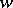on a complex manifoldis a couplewhere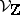is a locally constant sheaf of finitely-generated Abelian groups on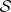, and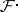is a finite decreasing filtration of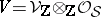by holomorphic subbundles, subject to the following conditions: i) the flat connectionondefined by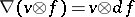, for,local sections ofand, respectively, satisfies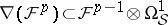(Griffiths' transversality); ii) for each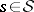, the pair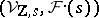is a Hodge structure of weight.
A polarization of a variation of Hodge structure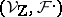is a flat bilinear form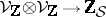which induces a polarization of the Hodge structure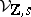for each. Similar notions exist forreplaced byor, [a2]. If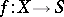is a proper smooth morphism of algebraic varieties over, then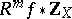is the underlying local system of a polarizable variation of Hodge structure on. By a result of A. Borel, for a polarized variation of Hodge structure on a complex manifoldof the form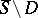, where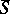is compact and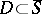is a divisor with normal crossings, the monodromy around each local component ofis quasi-unipotent [a3] (monodromy theorem). A polarized variation of Hodge structure overgives rise to a holomorphic period mapping fromto a classifying space of Hodge structures (see Period mapping).
If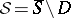witha compact Kähler manifold anda divisor with normal crossings on, then for a polarized variation of Hodge structureon, the sheafhas a minimal extension to a perverse sheaf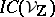onandcarries a pure Hodge structure [a4][a6]. In fact,is part of a polarized Hodge module [a7]. Generalizations are the notions of variation of mixed Hodge structure [a8], [a9] and mixed Hodge module [a10].